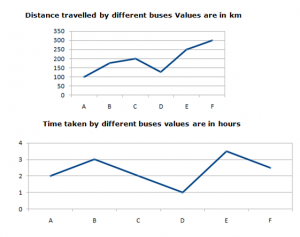## Data Interpretation : Set 02

Direction: 1-5): Given below in the table showing data of a metro travelling from Mumbai to Goa:
Note: Speed = Distance/ Time

 Station Name Distance(In Km) Time Taken(In hours) Speed(In kmph) MUMBAI-PUNE – 8 – JAIPUR-GOA 1150 – – DELHI-PUNE – 9 118.8 MUMBAI-DELHI 950 12 – PUNE – GOA – – 133.3

1) If the distance between Pune to Goa is 150km less than the Jaipur to Goa Then what will be the approximate time taken to travel Pune – Goa?
a) 6.5hr

b) 8.4hr

c) 7.5hr

d) 9.5hr

e) Cannot be determined

c) 7.5hr

Distance between Jaipur to Goa = 1150

Distance between Pune to Goa = 1150-150=1000

Time taken =  1000/133.3=~7.5

2) What will be the speed of train from Jaipur-Goa ,if the time taken to travel Jaipur -Goa 5hr less then the average of time taken from Mumbai-Delhi and Mumbai-Pune together?

a) 238km/hr

b) 260m/hr

c) 280km/sec

d) 230km/hr

e) None of these

d) 230 km/hr

Average time taken=12+8=20/2=10

Time taken to travel Jaipur – Goa=10 – 5=5

Speed =1150/5= 230 km/hr

3) If the speed of the train maintained from Mumbai – Delhi below the speed of train Delhi-Pune what will be average speed of Mumbai – Delhi and Delhi – Pune?

a) 220km/hr

b) 130km/hr

c) 250km/hr

d) Cannot be determined

e) None of these

d) Cannot be determined

speed of the train is not  mentioned  from Mumbai – Delhi.

So we cannot find average speed.

4) Average distance travelled by the train from all the station is 1114 then what will be total distance covered from Delhi-Pune , Pune – Goa and Mumbai to Pune?

a) 3200 km

b) 3470 km

c) 3800 km

d) 2920 km

e)3310 km

b) 3470 km

Total distance travelled by train from all the station = 1114×5=5570

Remaining Distance = 5570-2100 = 3470 km

5) What will be the speed of the train from Mumbai-Pune If it halts 20 min for every 2 hours of journey and its distance is 450 km more than Mumbai-Delhi?

a) 175.5km/hr

b) 155.5km/hr

c) 185km/hr

d) 165km/hr

e) 135km/hr

b) 155.5km/hr

Total time taken to travel form Mumbai to Pune = 8 hrs

Due to halt 20 mins for every 2hrs = 9 hrs to complete the journey

Distance between  Mumbai to Pune= 950 + 450= 1400

speed =  1400/9= 155.5 km/hr

Direction 6-10): Study the following chart carefully and answer the questions below:6)  What is the approximate average speed of buses A,B and C?

a) 53.72 km/hr

b) 54.25 km/hr

c) 60.2 km/hr

d) 53.5 km/hr

e) 67.85 km/hr

e) 67.85 km/hr
Average speed = (Total distance)/( Total time taken)

=  475/7=67.85km

7)  Due to stoppages if bus F runs 100km/hr. What would be delayed by stoppages (minutes)?

a) 10minutes

b) 45minutes

c) 25minutes

d) 1hr 15minutes

e) 30minutes

e) 30minutes

In Normal time taken by bus F = t = 2hr 30 min

Due to stoppages time taken by bus F =300/100= 3hr

required delay time = 30 min

8)  What is the approximate average distance traveled by all bus?

a) 180km

b) 192km

c) 190km

d) 189km

e) 195km

b) 192km

Required average =  (100+175+200+125+250+300)/6= 191.6 km

9)  Find the ratio of the speed of bus D and F?

a) 24:25

b) 25:24

c) 12:5

d) 10:14

e) 5:6

Required ratio is
125/1:300/2.5=120 : 120=25 : 24

10)  In Which of the following buses runs at maximum speed?

a) E

b) F

c) D

d) B

e) Other than given options

c) D

Speed of buses

A = 50 km/hr

B= 55 km/hr

C= 100 km/hr

D= 125km/hr

E=  (250×2)/7= 71.3km/hr

F = 120 km/hr

bus D is runs at maximum speed